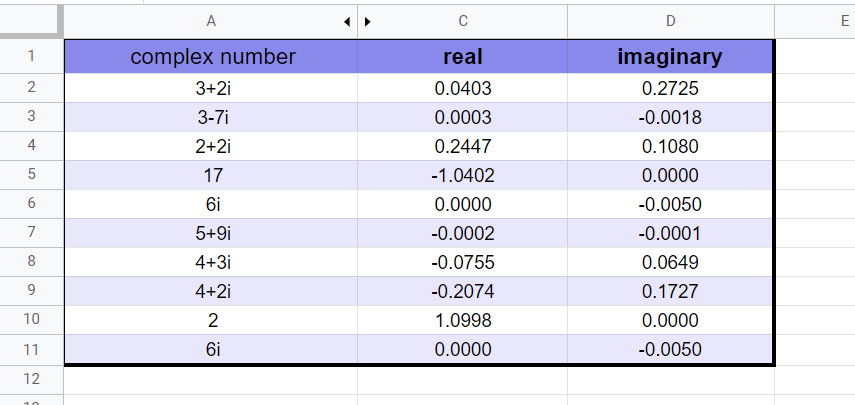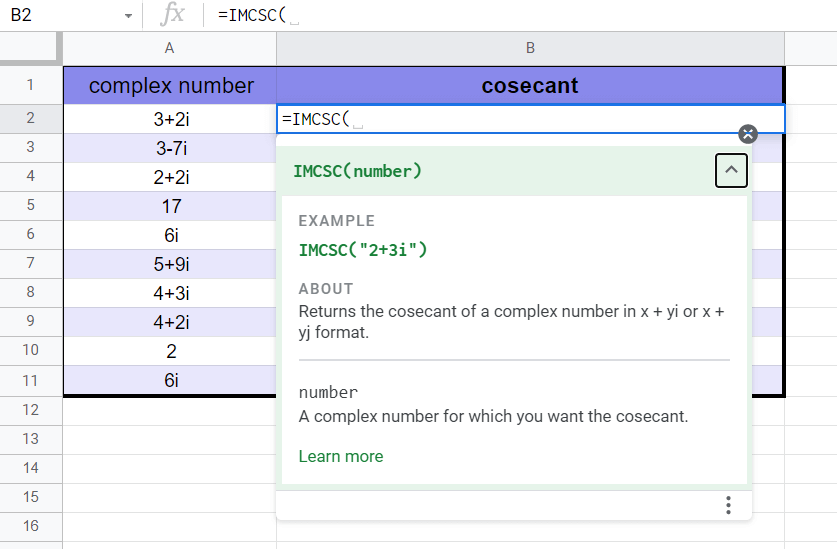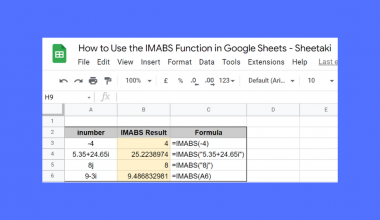How To Use IMCSC Function in Google Sheets

The IMCSC function in Google Sheets is useful when you need to return the cosecant of a particular complex number.

Complex numbers require specialized functions like IMCSC since regular mathematical functions cannot deal with input in the form a + bi or a + bj.

The rules for using the IMCSC function in Google Sheets are as follows:

• The function accepts a single argument which is the complex number we would like to obtain the cosecant of.
• The function then returns the cosecant of the provided complex number.
• The cosecant of a complex number is simply the reciprocal of its sine function.

Let’s look into how we can use this function with a brief use case!

Complex numbers are made up of two coefficients: the real coefficient and the imaginary coefficient. The horizontal axis is the real axis, and the vertical axis is the imaginary axis, forming a 2D plane. The coefficients allow us to use trigonometric functions within this plane.

In this example, we have a sample dataset of complex numbers. We would like to return various trigonometric values for each complex number, including its cosecant. Using the IMCSC function, we can easily return the cosecant of each complex number in the dataset.

Now that we know when to use the IMCSC function let’s dive into how to use it and work on an actual sample spreadsheet.

The Anatomy of the IMCSC Function

So the syntax (the way we write) of the IMCSC function is as follows:

=IMCSC(number)

Let’s dissect this thing and understand what each of these terms means:

• = the equal sign is how we start any function in Google Sheets.
• IMCSC() is our IMCSC function. It computes the cosecant of a given complex number
• number is the complex number which we would like to return the cosecant of. It usually comes in the a+bi or a+bj format.

A Real Example of Using IMCSC Function

Let’s look at an actual example of the IMCSC function being used in a Google Sheet spreadsheet.

In the screenshot below, we have a data set of complex numbers along with their corresponding cosecant values.To get the values in Column B, we just need to use the following formula:

=IMCSC(A2)

You can make a copy of the spreadsheet above using the link I have attached below.

We have another example seen below. In this table, we have the same dataset but we also use additional functions to retrieve the real and imaginary coefficients of the cosecant. The IMAGINARY and IMREAL functions are useful when you need to get these coefficients.Now that you’ve seen the IMCSC function in Google Sheets in action, let’s start learning how to write the function ourselves!

How to Use IMCSC Function in Google Sheets

1. First, we need to prepare our input. In the table below, we have our complex numbers in the first column of our worksheet.2. To start using the IMCSC function, first select the cell, we will first put our function’s output. In this example, we’ll be starting with cell B2.
3. Next, we just have to type the equal sign ‘=‘ to begin the function, followed by ‘IMCSC(‘.
4. As seen below, a tooltip box appears with info on the IMCSC function. We can click on the arrow on the top-right-hand corner of the box to minimize it if necessary.5. Next, we’ll input the argument for our function. In this case, cell A2 is the location of our first complex number.
Afterward, simply hit Enter on your keyboard to let the function return the cosecant.6. Finally, drag down the formula in cell B2 to fill out the rest of the column.1. Why does my formula output a #NUM! error?
A #NUM! error indicates that the given arguments are invalid and prevents the calculation from evaluating. For the IMCSC function, the formula returns a #NUM! error if the complex number doesn’t have lower case i or j as their variable for the imaginary coefficient.

That’s all you need to know to start using the IMCSC function in Google Sheets. After going through this step-by-step guide, you should now know how to use the IMCSC function in Google Sheets. It will come in handy whenever you need to find the cosecant of complex numbers.

You can now use the IMCSC functions in Google Sheets together with the various other Google Sheets formulas available to create amazing worksheets.

Stay notified of new Google Sheets guides like this by subscribing to our newsletter!Our goal this year is to create lots of rich, bite-sized tutorials for Google Sheets users like you. If you liked this one, you'd love what we are working on! Readers receive ✨ early access ✨ to new content.

You May Also LikeHow to Use INDIRECT Function in Google Sheets

The INDIRECT function in Google Sheets is useful if you want to return a cell reference specified by…How to Use DATEVALUE Function in Google Sheets

The DATEVALUE function in Google Sheets is a simple function used to return the number that represents the…How to Use AVERAGE.WEIGHTED Function in Google Sheets

The AVERAGE.WEIGHTED function in Google Sheets is useful when trying to find the average of a data set…How to Get Subscript and Superscript Values in Google Sheets

A few days back I was making some changes and adding data into a Google Sheet as part…